# NCERT Solutions for Class 6 Maths Chapter 3 Playing with Numbers

In this page, you will find Chapter 3 Playing with Numbers Class 6 Maths NCERT Solutions that will develop your problem solving skills and help in completing homework on time. These NCERT Solutions will serve as beneficial tool that can be used to recall various questions any time. Also you can Download PDF of Chapter 3 Playing with Numbers NCERT Solutions Class 6 Maths to practice in a better way.

Through these NCERT Solutions, students can cross check their answers and also whether they learned it properly or not. These solutions are updated according to the latest NCERT Class 9 Maths textbook. It will make aware of the difficulty of questions and learning efficiently.Page No: 50

Exercise 3.1

1. Write all the factors of the following numbers :
(a) 24
(b) 15
(c) 21
(d) 27
(e) 12
(f) 20
(g) 18
(h) 23
(i) 36

(a) 1 × 24 = 24
2 × 12 = 24
3 × 8 = 24
4 × 6 = 24
6 × 4 = 24
8 × 3 = 24
12 × 2 = 24
24 = 24 × 1
1,2,3,4,6,8,12 and 24 are the factors of 24.

(b) 1 × 15 =15
3 × 5 = 15
5 × 3 = 15
15 × 1 = 15
1,3,5 and 15 are the factors of 15.

(c) 1× 21 = 21
3 × 7 = 21
7 × 3 = 21
1, 3 and 7 are the factors of 21

(d) 1 × 27 = 27
3 × 9 = 27
9 × 3 = 27
1, 27, 3 and 9 are the factors of 27

(e) 1 × 12 = 12
2 × 6 = 12
3 × 4 = 12
4 × 3 = 12
6 × 2 = 12
12 × 1 = 12
1, 2,3,4,6 and 12 are the factors of 12.

(f) 1 × 20 =20
2 × 10 = 20
4 × 5 = 20
5 × 4 = 20
10 × 2 = 20
20 × 1 = 20
1, 2, 4, 5, 10 and 20 are the factors of 20.

(g) 1 × 18 = 18
2 × 9 = 18
3 × 6 = 18
6 × 3 = 18
9 × 2 = 18
1,2,3,6 and 9 are the factors of 18.

(h) 1 × 23 = 23
23 × 1 = 23
1 and 23 are the factors of 23.

(i) 1 × 36 =36
2 × 18 = 36
3 × 12 = 36
4 × 9 = 36
6 × 6 = 36
9 × 4 =36
12 × 3 = 36
18 × 2 = 36
36 × 1 = 36
1,2,3,4,6,9,12,18 and 36 are the factors of 36.

2. Write first five multiples of :
(a) 5
(b) 8
(c) 9

(a) Five multiples of 5 are
5 × 1 = 5
5 × 2 = 10
5 × 3 = 15
5 × 4 = 20
5 × 5 =25

(b) Five multiples of 8 are
8 × 1 = 8
8 × 2 = 16
8 × 3 = 24
8 × 4 = 32
8 × 5 = 40

(c) Five multiples of 9
9 × 1 = 9
9 × 2 = 18
9 × 3 = 27
9 × 4 = 36
9 × 5 = 45

3. Match the items in column 1 with the items in column 2.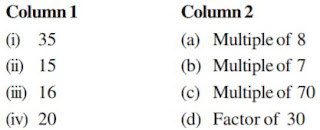(i) → (b) Multiple of 7
(ii) → (d) Factor of 30
(iii) → (a) Multiple of 8
(iv) → (f) Factor of 20
(v) → (e) Factor of 50

Page No: 51

4. Find all the multiples of 9 up to 100.

9 × 1 = 9, 9 × 2 = 18,  9 × 3 = 27,  9 × 4 = 36,  9 × 5 = 45, 9 × 6 = 54,  9 × 7 = 63 ,9 × 8 = 72,  9 × 9 = 81,  9 × 10 = 90, 9 × 11 = 99
Multiples of 9 up to 100 are: 9, 18, 27, 36, 45, 54, 63, 72, 81, 90, and 99

Page No: 53

Exercise 3.2

1. What is the sum of any two
(a) Odd numbers
(b) Even numbers

(a) 11 + 13 = 24
Sum of two odd number is always even.

(b) 12 + 14 = 26
Sum of two even number is always even.

2. State whether the following statements are True or False:
(a) The sum of three odd numbers is even.
(b) The sum of two odd numbers and one even number is even.
(c) The product of three odd numbers is odd.
(d) If an even number is divided by 2, the quotient is always odd.
(e) All prime numbers are odd.
(f) Prime numbers do not have any factors.
(g) Sum of two prime numbers is always even.
(h) 2 is the only even prime number.
(i) All even numbers are composite numbers.
(j) The product of two even numbers is always even.

(a) 5+7+9 = 21 (false)
(b) 5+7+8 = 20 (true)
(c) 3 × 5 × 7 = 105 (true)
(d) 4 ÷ 2 = 2 (false)
(e) 2 is a prime number and it is also even
(f) false
(g) false
(h) true
(i) false
(j) true

3. The numbers 13 and 31 are prime numbers. Both these numbers have same digits 1 and 3. Find such pairs of prime numbers up to 100.

17, 71
37, 23
79, 97

4. Write down separately the prime and composite numbers less than 20.

Prime numbers which are less than 20:
2, 3, 5, 7, 11, 13, 17, 19

Composite numbers which are less than 20:
4, 6, 8, 9, 10, 12, 14, 15, 16, 18

5. What is the greatest prime number between 1 and 10?

1 and 10 prime number are 2, 3, 5, and 7.
7 is the greatest.

6. Express the following as the sum of two odd primes.
(a) 44
(b) 36
(c) 24
(d) 18

(a) 37 + 7 = 44
(b) 31 + 5 = 36
(c) 19 + 5 = 24
(d) 11 + 7 = 18

7. Give three pairs of prime numbers whose difference is 2.
[Remark : Two prime numbers whose difference is 2 are called twin primes].

3, 5
41, 43
71, 73

8. Which of the following numbers are prime?
(a) 23
(b) 51
(c) 37
(d) 26

(a) 23 = 1 × 23 = 23 , 23 × 1 = 23

(b) 51 = 1 × 51 = 51 , 3 × 17 = 51, 17 × 3 = 51 , 51 × 1 = 51
1,3,17,51 they are not prime number.

(c) 37 = 1 × 37 = 37 , 37 × 1 = 37. They are prime number.

(d) 26 = 1 × 26 = 26, 2 × 13 = 26, 13 × 2 = 26, 26 × 1 = 26. They are not prime number.

9. Write seven consecutive composite numbers less than 100 so that there is no prime number between them.

90 , 91 , 92 , 93 , 94 ,95 and 96 are seven consecutive composite number less than 100 and there is no prime number between them.

Page No: 54

10. Express each of the following numbers as the sum of three odd primes:
(a) 21
(b) 31
(c) 53
(d) 61

(a) 3 + 7 + 11 = 21
(b) 5 + 7 + 19 = 31
(c) 3 + 19 +31 = 53
(d) 11 + 19 + 31 = 61

11. Write five pairs of prime numbers less than 20 whose sum is divisible by 5. (Hint : 3+7 = 10)

(i) 2 + 3 =5
(ii) 2 + 13 = 15
(iii) 3 + 17 = 20
(iv) 7 +13 = 20
(v) 19 + 11 = 30

12.Fill in the blanks :
(a) A number which has only two factors is called a ______.
(b) A number which has more than two factors is called a ______.
(c) 1 is neither ______ nor ______.
(d) The smallest prime number is ______.
(e) The smallest composite number is _____.
(f) The smallest even number is ______.

(a) Prime number
(b) Composite number
(c) Prime number
(d) 2
(e) 4
(f) 2

Page No: 57

Exercise 3.3

1. Using divisibility tests, determine which of the following numbers are divisible by 2; by 3; by 4; by 5; by 6; by 8; by 9; by 10 ; by 11 (say, yes or no):2. Using divisibility tests, determine which of the following numbers are divisible by 4; by 8:
(a) 572
(b) 726352
(c) 5500
(d) 6000
(e) 12159
(f) 14560
(g) 21084
(h) 31795072
(i) 1700
(j) 2150

(a) 572→ Last two digit is divisible by 4 , so the given three digit is divisible by 4 but not divisible by 8.

(b) 726352 → Last two digit is divisible by 4 , so the given number is divisible by 4 and last three digit is also divisible by 8 so the given number is divisible by 8.

(c) 5500 → Last two digit is divisible by 4, but last three digit number is not divisible by 8.

(d) 6000 → Last two digit is divisible by 4, but last three digit number is not divisible by 8.

(e) 12159 → Last two digit is not divisible by 4, or last three digit number is also not divisible by 8.

(f) 14560 → Last two digit is divisible by 4 and last three digit is also divisible by 8.

(g) 21084 → Last two digit is divisible by 4 and last three digit is not divisible by 8.

(h) 31795072 → Last two digit is divisible by 4 and last three digit is divisible by 8.

(i) 1700 → Last two digit is divisible by 4, and last three digit is not divisible by 8.

(j) 2150 → Last two digit is not divisible by 4, or last three digit number is also not divisible by 8.

3. Using divisibility tests, determine which of following numbers are divisible by 6:
(a) 297144
(b) 1258
(c) 4335
(d) 61233
(e) 901352
(f) 438750
(g) 1790184
(h) 12583
(i) 639210
(j) 17852

(i) 297144 → last digit of the number is 4 which is divisible by 2.
Sum of all digits = 27.
27 is divisible by 3, so the given number is also divisible by 3.
Both the number is divisible by 2 and 3.
So, the given number is divisible by 6.

(ii) 1258 → last digit of the number is 8 which is divisible by 2.
Sum of all digits =16.
16 is not divisible by 3, so the given number is also not divisible by 3.
So the number is not divisible by both 2 and 3, or it is not divisible by 6.

(iii) 4335 → last digit of the number is 5, which is not divisible by 2.
So, the given number is also not divisible by 2.
Sum of all digits = 15
Since 15 is divisible by 3, so the given number is also divisible by 3.
So, the number is not divisible by both 2 and 3, or it is not divisible by 6.

(iv) 61233 → last digit of the number is 3, which is not divisible by 2.
Sum of all digits = 15.
15 is divisible by 3, so the given number is also divisible by 3.
So, the number is not divisible by both 2 and 3, it is not divisible by 6.

(v) 901352 = last digit of the number is 2 which is divisible by 2.
Sum of all digits = 20.
20 is not divisible by 3, so the given number is also not divisible by 3.
So, the number is not divisible by both 2 and 3, it is not divisible by 6.

(vi) 438750 = last digit of the number is 0 which is divisible by 2.
Sum of all digits = 27.
27 is divisible by 3, so the given number is also divisible by 3.
So, the number is divisible by both 2 and 3, it is divisible by 6.

(vii) 1790184 = last digit of the number is 4 which is divisible by 2.
Sum of all digits = 30
Since 30 is divisible by 3, the given number is also divisible by 3.
So, the number is divisible by both 2 and 3, it is divisible by 6.

(viii) 12583 = last digit of the number is 3 which is not divisible by 2.
Sum of all digits = 19.
19 is not divisible by 3, the given number is also not divisible by 3.
So, the number is not divisible by both 2 and 3, it is not divisible by 6.

(ix) 639210 = last digit of the number is 0 which is divisible by 2.
Sum of all digits = 21.
21 is divisible by 3, the given number is also divisible by 3.
Sp, the number is divisible by both 2 and 3, it is divisible by 6.

(x) 17852 =  last digit of the number is 2 which is divisible by 2.
Sum of all digits = 23.
Since 23 is not divisible by 3, the given number is also not divisible by 3.
So, the number is not divisible by both 2 and 3, it is not divisible by 6.

4. Using divisibility tests, determine which of the following numbers are divisible by 11:
(a) 5445
(b) 10824
(c) 7138965
(d) 70169308
(e) 10000001
(f) 901153

(a) 5445 = Sum of the digits at odd places = 5 + 4 = 9
Sum of the digits at even places = 4 + 5 = 9
Difference = 9 − 9 = 0
Difference between the sum of the digits at odd places and the sum of the digits at even places is 0, So, 5445 is divisible by 11.

(b) 10824 = Sum of the digits at odd places = 4 + 8 + 1 = 13
Sum of the digits at even places = 2 + 0 = 2
Difference = 13 − 2 = 11
The difference between the sum of the digits at odd places and the sum of the digits at even places is 11.
So, 10824 is divisible by 11.

(c) 7138965 = Sum of the digits at odd places = 5 + 9 + 3 + 7 = 24
Sum of the digits at even places = 6 + 8 + 1 = 15
Difference = 24 − 15 = 9
Difference between the sum of the digits at odd places and the sum of digits at even places is 9, which is not divisible by 11.
So, 7138965  is not divisible by 11.

(d) 70169308 = Sum of the digits at odd places = 8 + 3 + 6 + 0 = 17
Sum of the digits at even places = 0 + 9 + 1 + 7 = 17
Difference = 17 − 17 = 0
Difference between the sum of the digits at odd places and the sum of the digits at even places is 0.
So, 70169308 is divisible by 11.

(e) 10000001 = Sum of the digits at odd places = 1
Sum of the digits at even places = 1
Difference = 1 − 1 = 0
Difference between the sum of the digits at odd places and the sum of the digits at even places is 0.
So, 10000001 is divisible by 11.

(f) 901153 = Sum of the digits at odd places = 3 + 1 + 0 = 4
Sum of the digits at even places = 5 + 1 + 9 = 15
Difference = 15 − 4 = 11
Difference between the sum of the digits at odd places and the sum of the digits at even places is 11, which is divisible by 11.
So, 901153 is divisible by 11.

5. Write the smallest digit and the greatest digit in the blank space of each of the following numbers so that the number formed is divisible by 3:
(a) _____ 6724
(b) 4765 ____ 2

(a) _____ 6724
Sum of the remaining digits = 19
To make the number divisible by 3, the sum of its digits should be divisible by 3.
The smallest multiple of 3 which comes after 19 is 21.
Therefore, smallest number = 21 − 19 = 2
Now, 2 + 3 + 3 = 8
However, 2 + 3 + 3 + 3 = 11
If we put 8, then the sum of the digits will be 27 and as 27 is divisible by 3, the number will also be divisible by 3.
Therefore, the largest number is 8.

(b) 4765_____2
Sum of the remaining digits = 24
To make the number divisible by 3, the sum of its digits should be divisible by 3. As 24 is already divisible by 3, the smallest number that can be placed here is 0.
Now, 0 + 3 = 3
3 + 3 = 6
3 + 3 + 3 = 9
However, 3 + 3 + 3 + 3 = 12
If we put 9, then the sum of the digits will be 33 and as 33 is divisible by 3, the number will also be divisible by 3.
Therefore, the largest number is 9.

Page No: 58

6. Write a digit in the blank space of each of the following numbers so that the number formed is divisible by 11 :
(a) 92 ____ 389
(b) 8 ____ 9484

(a) 92 ____ 389
Sum of the digits at odd places = 9 + 3 + 2 = 14
Sum of the digits at even places = 8 + a + 9 = 17 + a
Difference = 17 + a − 14 = 3 + a
For a number to be divisible by 11, this difference should be zero or a multiple of 11.
If 3 + a = 0, then a = −3
However, it cannot be negative.

A closest multiple of 11 which is near to 3 has to be taken. It is 11 itself.
3 + a = 11
a = 8
Therefore, the required digit is 8.

(b) 8 ____ 9484
Sum of the digits at odd places = 4 + 4 + a = 8 + a
Sum of the digits at even places = 8 + 9 + 8 = 25
Difference = 25 − (8 + a) = 17 − a

For a number to be divisible by 11, this difference should be zero or a multiple of 11.
If 17 − a = 0, then, a = 17
This is not possible.

Page No: 59

Exercise 3.4

1. Find the common factors of :
(a) 20 and 28
(b) 15 and 25
(c) 35 and 50
(d) 56 and 120

(a) Factors of 20 = 1, 2, 4, 5, 10, 20
Factors of 28 = 1, 2, 4, 7, 14, 28
Common factors = 1, 2, 4

(b) Factor of 15 = 1, 3, 5, 15
Factor of 25 = 1, 5, 25
Common factors =1, 5

(c) Factor of 35 = 1, 5, 7, 35
Factor of 50 = 1, 2, 5, 10, 25, 50
Common factors = 1, 5

(d) Factors of 56 = 1, 2, 4, 7, 8, 14, 28, 56
Factors of 120 = 1, 2, 3, 4, 5, 6, 8, 10, 12, 15, 20, 24, 30, 40, 60, 120
Common factors = 1, 2, 4, 8

2. Find the common factors of :
(a) 4, 8 and 12
(b) 5, 15 and 25

(a) Factors of 4 = 1, 2, 4
Factors of 8 = 1, 2, 4, 8
Factors of 12 = 1, 2, 3, 4, 6, 12
Common factors = 1, 2, 4

(b) 5, 15, and 25
Factors of 5 = 1, 5
Factors of 15 = 1, 3, 5, 15
Factors of 25 = 1, 5, 25
Common factors = 1, 5

3. Find first three common multiples of :
(a) 6 and 8
(b) 12 and 18

(a) 6 = 6, 12, 18, 24, 30  and  8 = 8, 16, 24, 32, 40
(b) 12 = 12, 24, 36, 48, 60 and 18 = 18, 36, 54, 72, 90

4. Write all the numbers less than 100 which are common multiples of 3 and 4.

Multiple of 3 = 3, 6, 9, 12, 15
Multiple of 4 = 4, 8, 12, 16, 20

5. Which of the following numbers are co-prime?
(a) 18 and 35
(b) 15 and 37
(c) 30 and 415
(d) 17 and 68
(e) 216 and 215
(f) 81 and 16

(a) Factors of 18 = 1, 2, 3, 6, 9, 18
Factors of 35 = 1, 5, 7, 35
Common factor = 1
Its common factor is 1 so it is co-prime.

(b) Factor of 15 = 1, 3, 5, 15
Factor of 37 = 1 and 37
Common factor = 1
Its common factor is 1 so it is co-prime.

(c) Factor of 30 = 1, 2, 3, 5, 6, 10, 15, 30
Factor of 415 = 1, 5, 83, 415
Common factor = 1 and 5
Its common factor are more than 1. So it is not co-prime.

(d) Factor of 17 = 1, 17
Factor of 68 = 1, 2, 4, 17, 34, 68
Common factor = 1 and 17
Its common factor are more than 1. So it is not co-prime.

(e) Factor of  216 = 1, 2, 3, 4, 6, 8, 9, 12, 18, 24, 27, 36, 54, 72, 108, 216
Factor 215 = 1, 5, 43, 215
Common factor = 1
Its common factor is 1 so it is co-prime.

(f) Factor of 81 = 1, 3, 9, 27, 81
Factor of 16 = 1, 2, 4, 8, 16
Common factor = 1
Its common factor is 1 so it is co-prime.

6. A number is divisible by both 5 and 12. By which other number will that number be always divisible?

Factor of 5 = 1,5
Factor of 12 = 1, 2, 3, 4, 6, 12
Common factor = 1
And the product of these two number is 60.
Factor of 60 = 1, 2, 3, 4, 5, 6, 10, 12, 15, 20, 30, 60.

7. A number is divisible by 12. By what other numbers will that number be divisible?

Factor of 12  =1, 2, 3, 4, 6, 12.
Clearly, 1, 2, 3, 4, and 6 are numbers other than 12 by which this number is also divisible.

Page No: 61

Exercise 3.5

1. Which of the following statements are true?
(a) If a number is divisible by 3, it must be divisible by 9.
(b) If a number is divisible by 9, it must be divisible by 3.
(c) A number is divisible by 18, if it is divisible by both 3 and 6.
(d) If a number is divisible by 9 and 10 both, then it must be divisible by 90.
(e) If two numbers are co-primes, at least one of them must be prime.
(f) All numbers which are divisible by 4 must also be divisible by 8.
(g) All numbers which are divisible by 8 must also be divisible by 4.
(h) If a number exactly divides two numbers separately, it must exactly divide their sum.
(i) If a number exactly divides the sum of two numbers, it must exactly divide the two numbers separately.

(a) False 12 is divisible by 3 but not divisible by 9.
(b) True 18 is divisible by 9 it also divisible by 3.
(c) False 30 is divisible by both 3 and 6 but not divisible by 18.
(d) True
(e) False
(f) False
(g) True
(h) True
(i) False

Page No: 62

2.Here are two different factor trees for 60. Write the missing numbers.(a) 6 = 2 × 3
10 = 5 × 2

(b) 60 = 30 × 2
30 = 10 × 3
10 = 5 × 2

3. Which factors are not included in the prime factorisation of a composite number?

1 is not included in the prime factorisation of a composite number because it is the factor for all numbers.

4. Write the greatest 4-digit number and express it in terms of its prime factors.

Greatest 4-digit number = 9999
Prime factors of 9999 = 3 × 3 × 11 × 101 = 9999

5. Write the smallest 5-digit number and express it in the form of its prime factors.

Smallest 5–digit number = 10,000
Prime factors of   10,000 = 2 × 2 × 2 × 2 × 5 × 5 × 5 × 5 = 10,000

6. Find all the prime factors of 1729 and arrange them in ascending order. Now state the relation, if any; between two consecutive prime factors.

1729 = 7 × 13 × 19
13 – 7 = 6
19 – 3 = 6
Difference between of two consecutive prime factor is 6.

7. The product of three consecutive numbers is always divisible by 6. Verify this statement with the help of some examples.

24 = 2 × 3 × 4 is divisible by 6
990 = 9 × 10 × 11 = is divisible by 6
9240 = 20 × 21 × 22 is divisible by 6

8. The sum of two consecutive odd numbers is divisible by 4. Verify this statement with the help of some examples.

3 + 5 = 8 is divisible by 4
15 + 17 = 32 is divisible by 4
19 + 21 = 40 is divisible by 4

9. In which of the following expressions, prime factorisation has been done?
(a) 24 = 2 × 3 × 4
(b) 56 = 7 × 2 × 2 × 2
(c) 70 = 2 × 5 × 7
(d) 54 = 2 × 3 × 9

(a) 24 = 2 × 3 × 4
In this prime factorization, 4 is composite number , because it is divisible by 1, 2, 4.

(b) 56 = 7 × 2 × 2 × 2
In this  prime factorization, all are prime number because they are divided by 1 and itself.

(c) 70 = 2 × 5 × 7
In this factors, all are prime number because they are divided by 1 and itself.

(d) 54 = 2 × 3 × 9
In this prime factorization, 9 is composite number , because it is divisible by 1, 3, 9.

10. Determine if 25110 is divisible by 45.
[Hint : 5 and 9 are co-prime numbers. Test the divisibility of the number by 5 and 9].

Factors of 5 = 1, 5
Factors of 9 = 1, 3, 9
45 = 5 × 9
25110 last digit is 0, it is divisible by 5.
Sum of the digits 25110 = 2 + 5 + 1 + 1 + 0 = 9
25110 is divisible by 9
So , the number is divisible by both 5 and 9, it is divisible by 45.

11. 18 is divisible by both 2 and 3. It is also divisible by 2 × 3 = 6. Similarly, a number is divisible by both 4 and 6. Can we say that the number must also be divisible by 4 × 6 = 24? If not, give an example to justify your answer.

12 and 36 both are divisible by 4 and 6 both, but not divisible by 24.

12. I am the smallest number, having four different prime factors. Can you find me?

Product of 4 smallest prime numbers are .
2 × 3 × 5 × 7 = 210

Page No: 63

Exercise 3.6

1. Find the HCF of the following numbers :
(a) 18, 48
(b) 30, 42
(c) 18, 60
(d) 27, 63
(e) 36, 84
(f) 34, 102
(g) 70, 105, 175
(h) 91, 112, 49
(i) 18, 54, 81
(j) 12, 45, 75

(a)18 = 2 × 3 × 3
48 = 2 × 2 × 2 × 2 × 3
HCF = 2 × 3 = 6

(b)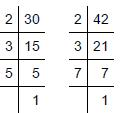30 = 2 × 3 × 5
42 = 2 × 3 × 7

HCF = 2 × 3 = 6
HCF of 30, 42 = 6

(c)27 = 3 × 3 × 3
63 = 3 × 3 × 7

HCF = 3 × 3 = 9
HCF of 27, 63 is 99

(d)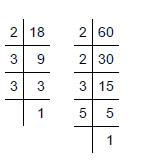18 = 2 × 3 × 3
60 = 2 × 2 × 3 × 5

HCF = 2 × 3 = 6
HCF of 18, 60 is 6

(e)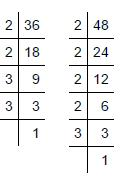36 = 2 × 2 × 3 × 3
84 = 2 × 2 × 3 × 7

HCF = 2 × 2 × 3 = 12
HCF of 36, 84 is 1212

(f)34 = 2 × 17
102 = 2 × 3 × 17

HCF = 2 × 17 = 34
HCF of 34, 102 is 34

(g)70 = 2 × 5 × 7
105 = 3 × 5 × 7
175 = 5 × 5 × 7

HCF = 5 × 7 = 35
HCF of 70, 105,17570, is 35

(h)91 = 7 × 13
112 = 2 × 2 × 2 × 2 × 7
49 = 7 × 7

HCF = 7
HCF of 91, 112, 49 is 7

(i)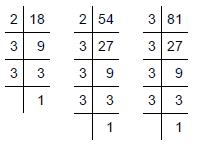18 = 2 × 3 × 3
54 = 2 × 3 × 3 × 3
81 = 3 × 3 × 3 × 3

HCF = 3 × 3 = 9
HCF of 18, 54, 81 is 99

j)12 = 2 × 2 × 3
45 = 3 × 3 × 5
75 = 3 × 5 × 5

HCF = 3
HCF of 12,45,75 is 33.

2. What is the HCF of two consecutive
(a) numbers?
(b) even numbers?
(c) odd numbers?

(a) 1 e.g., HCF of 2 and 3 is 1.
(b) 2 e.g., HCF of 2 and 4 is 2.
(c) 1 e.g., HCF of 3 and 5 is 1.

Page No: 64

3. HCF of co-prime numbers 4 and 15 was found as follows by factorisation : 4 = 2 × 2 and 15 = 3 × 5 since there is no common prime factor, so HCF of 4 and 15 is 0. Is the answer correct? If not, what is the correct HCF?

No, 1 is the correct answer.

Page No: 67

Exercise 3.7

1. Renu purchases two bags of fertiliser of weights 75 kg and 69 kg. Find the maximum value of weight which can measure the weight of the fertiliser exact number of times.

Weight of bags are 75 kg and 69 kg75 = 3 × 5 × 5
69 = 3 × 23
HCF = 3
Hence, the maximum value of weight, which can measure the weight of the fertilizer exact number of times, = 3 kg.

2. Three boys step off together from the same spot. Their steps measure 63 cm, 70 cm and 77 cm respectively. What is the minimum distance each should cover so that all can cover the distance in complete steps?

Step measure by first boy = 63 cm
Step measure by second boy = 70 cm
Step measure by third boy = 77 cmLCM = 2 × 3 × 3 × 5 × 7 × 11 = 6930
The minimum distance each should cover so that all can cover the distance in complete steps is 6930 cm.

3. The length, breadth and height of a room are 825 cm, 675 cm and 450 cm respectively. Find the longest tape which can measure the three dimensions of the room exactly.

Length  825 cm = 3 × 5 × 5 × 11
Breadth 675 cm = 3 × 3 × 3 × 5 × 5
Height 450 cm = 2 × 3 × 3 × 5 × 5
HCF of 825, 675, and 450 = 3 × 5 × 5 = 75 cm
Therefore, the longest tape is 75 cm.

4.Determine the smallest 3-digit number which is exactly divisible by 6, 8 and 12.LCM of 6,8,12 = 2 × 2 × 2 × 3 × = 24
Hence, the smallest 3-digit number which meets the requirements must be a multiple of 24.
The smallest 3 – digit multiple of 24 is 24× 5 = 120.

5. Determine the greatest 3-digit number exactly divisible by 8, 10 and 12.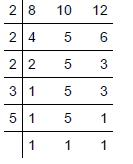LCM of 8,10, 12 = 2 × 2 × 2 × 3 × 5 =120

6.The traffic lights at three different road crossings change after every 48 seconds, 72 seconds and 108 seconds respectively. If they change simultaneously at 7 a.m., at what time will they change simultaneously again?LCM of 48, 72, 108 = 2 × 2 × 2 × 2 × 3 × 3 × 3 = 432

7.Three tankers contain 403 litres, 434 litres and 465 litres of diesel respectively. Find the maximum capacity of a container that can measure the diesel of the three containers exact number of times.403 = 13 × 31
434 = 2 × 7 × 31
465 = 3 × 5 × 31
HCF = 31

8. Find the least number which when divided by 6, 15 and 18 leave remainder 5 in each case.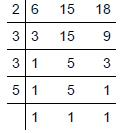LCM of 6, 15 , 18 = 2 × 3 × 3 × 5 = 90

9.Find the smallest 4-digit number which is divisible by 18, 24 and 32.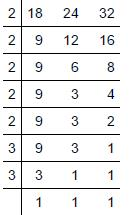LCM of 18 , 24 , 32 = 2 × 2 × 2 × 2 × 2 × 3 × 3 = 288

10.Find the LCM of the following numbers :
(a) 9 and 4
(b) 12 and 5
(c) 6 and 5
(d) 15 and 4
Observe a common property in the obtained LCMs. Is LCM the product of two numbers in each case?

(a) 9 and 4LCM = 2 × 2 × 3 × 3 = 36

(b) 12 and 5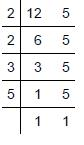LCM = 2 × 2 × 3 × 5 = 60

(c) 6 and 5LCM =2 × 3 × 5 = 30

(d) 15 and 4LCM = 2 × 2 × 3 ×  5 = 60
In each LCM multiple is 3.

11. Find the LCM of the following numbers in which one number is the factor of the other.
(a) 5, 20
(b) 6, 18
(c) 12, 48
(d) 9, 45
What do you observe in the results obtained?

Solution :

(a) 5, 20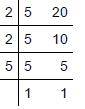LCM = 2 × 2 × 5 = 20

(b) 6, 18LCM = 2 × 3 × 3 = 18

(c) 12, 48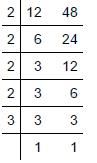LCM of 12 ,48 = 2 × 2 × 2 × 2 × 3 = 48

d) 9, 45LCM = 3 × 3 × 5 = 45

## NCERT Solutions for Class 6 Maths Chapter 3 Playing with Numbers

Chapter 3 NCERT Solutions will make you understand the topics in most simple manner. In the Chapter Playing with numbers, we will be dealing with factors and multiples, prime and composite numbers and divisibility tests.

• An exact divisor of a number is called its factor. The product of two numbers is called a multiple of each of the two numbers being multiplied.

• Numbers that have only two factors in the form of 1 and the number itself are called prime numbers. Numbers that have more than two factors are called composite numbers. The number 1 is neither a prime number nor a composite number.

• All numbers with 0, 2, 4, 6 or 8 in the unit's or one's place are multiples of 2, and are called even numbers. All numbers with 1, 3, 5, 7 or 9 in the unit's or one's place are called odd numbers.

Below we have provided exercisewise Class 6 NCERT Solutions which will be make much easier to memorize topics faster and frame better answers. It will help in building a great foundation of concepts and make easy for the students to understand basics.

Studyrankers experts have prepared these NCERT Solutions which will be help you in knowing variety of concepts and developing problem solving skills. It will prepare students to do better during immense pressure and at the same time make them fresh and enhances memory.

### NCERT Solutions for Class 6 Maths Chapters:

 Chapter 1 Knowing Our Numbers Chapter 2 Whole Numbers Chapter 4 Basic Geometrical Ideas Chapter 5 Understanding Elementary Shapes Chapter 6 Integers Chapter 7 Fractions Chapter 8 Decimals Chapter 9 Data Handling Chapter 10 Mensuration Chapter 11 Algebra Chapter 12 Ratio and Proportion Chapter 13 Symmetry Chapter 14 Practical Geometry

FAQ on Chapter 3 Playing with Numbers

#### How many exercises in Chapter 3 Playing with Numbers?

Chapter 3 Class 6 Maths NCERT Solutions consists of seven exercises which will be help in building a great foundation of concepts and make easy for the students to understand basics. It will increase concentration among students and help in revising the chapter properly.

#### What are co-prime numbers?

If the only common factor of two numbers is 1, then the two numbers are called co-prime numbers.

#### How to find the LCM of the numbers using the division method?

• Write the given numbers in a row.
• Divide the numbers by the smallest prime number that divides one or more of the given numbers.
• Write the number that is not divisible, in the second row.
• Write the new dividends in the second row.
• Divide the new dividends by another smallest prime number.
• Continue dividing till the dividends are all prime numbers or 1.
• Stop the process when all the new dividends are prime numbers or 1.

#### What are general rules of divisibility for all numbers?

If a number is divisible by another number, then it is also divisible by all the factors of the other number. If two numbers are divisible by another number, then their sum and difference is also divisible by the other number. If a number is divisible by two co-prime numbers, then it is also divisible by the product of the two co-prime numbers.